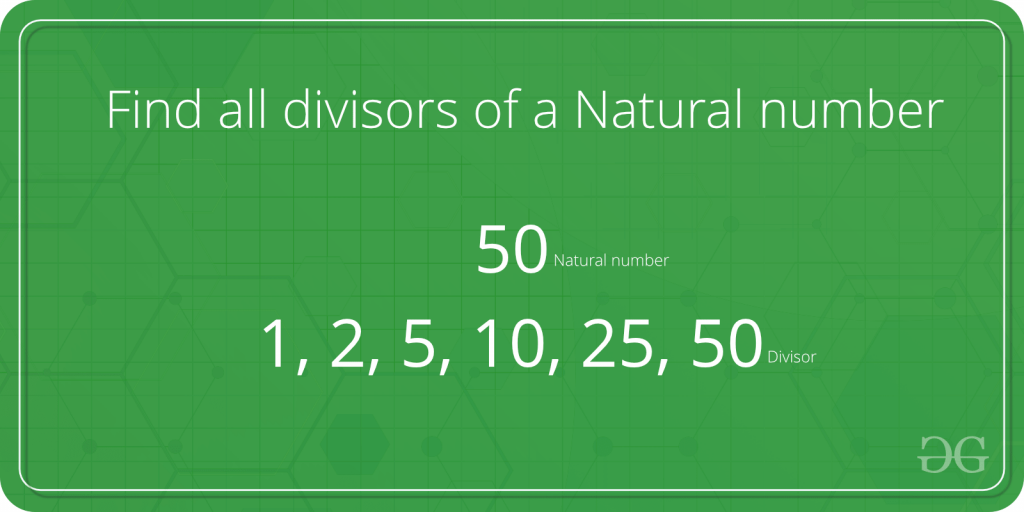Related Articles

# Find all factors of a natural number | Set 1

• Difficulty Level : Easy
• Last Updated : 06 Aug, 2021

Given a natural number n, print all distinct divisors of it.Attention reader! Don’t stop learning now. Get hold of all the important DSA concepts with the DSA Self Paced Course at a student-friendly price and become industry ready.  To complete your preparation from learning a language to DS Algo and many more,  please refer Complete Interview Preparation Course.

In case you wish to attend live classes with experts, please refer DSA Live Classes for Working Professionals and Competitive Programming Live for Students.

Examples:

``` Input : n = 10
Output: 1 2 5 10

Input:  n = 100
Output: 1 2 4 5 10 20 25 50 100

Input:  n = 125
Output: 1 5 25 125```

Note that this problem is different from finding all prime factors.

A Naive Solution would be to iterate all the numbers from 1 to n, checking if that number divides n and printing it. Below is a program for the same:

## C++

 `// C++ implementation of Naive method to print all``// divisors``#include ``using` `namespace` `std;` `// function to print the divisors``void` `printDivisors(``int` `n)``{``    ``for` `(``int` `i = 1; i <= n; i++)``        ``if` `(n % i == 0)``            ``cout <<``" "` `<< i;``}` `/* Driver program to test above function */``int` `main()``{``    ``cout <<``"The divisors of 100 are: \n"``;``    ``printDivisors(100);``    ``return` `0;``}` `// this code is contributed by shivanisinghss2110`

## C

 `// C implementation of Naive method to print all``// divisors``#include` `// function to print the divisors``void` `printDivisors(``int` `n)``{``    ``for` `(``int` `i=1;i<=n;i++)``        ``if` `(n%i==0)``            ``printf``(``"%d "``,i);``}` `/* Driver program to test above function */``int` `main()``{``    ``printf``(``"The divisors of 100 are: \n"``);``    ``printDivisors(100);``    ``return` `0;``}`

## Java

 `// Java implementation of Naive method to print all``// divisors` `class` `Test``{``    ``// method to print the divisors``    ``static` `void` `printDivisors(``int` `n)``    ``{``        ``for` `(``int` `i=``1``;i<=n;i++)``            ``if` `(n%i==``0``)``                ``System.out.print(i+``" "``);``    ``}` `    ``// Driver method``    ``public` `static` `void` `main(String args[])``    ``{``        ``System.out.println(``"The divisors of 100 are: "``);``        ``printDivisors(``100``);;``    ``}``}`

## Python

 `# Python implementation of Naive method``# to print all divisors` `# method to print the divisors``def` `printDivisors(n) :``    ``i ``=` `1``    ``while` `i <``=` `n :``        ``if` `(n ``%` `i``=``=``0``) :``            ``print` `i,``        ``i ``=` `i ``+` `1``        ` `# Driver method``print` `"The divisors of 100 are: "``printDivisors(``100``)` `#This code is contributed by Nikita Tiwari.`

## C#

 `// C# implementation of Naive method``// to print all divisors``using` `System;` `class` `GFG {``    ` `    ``// method to print the divisors``    ``static` `void` `printDivisors(``int` `n)``    ``{``        ``for` `(``int` `i = 1; i <= n; i++)``            ``if` `(n % i == 0)``                ``Console.Write( i + ``" "``);``    ``}` `    ``// Driver method``    ``public` `static` `void` `Main()``    ``{``        ``Console.Write(``"The divisors of"``,``                          ``" 100 are: "``);``        ``printDivisors(100);;``    ``}``}` `// This code is contributed by nitin mittal.`

## PHP

 ``

## Javascript

 ``

Output:

```The divisors of 100 are:
1 2 4 5 10 20 25 50 100```

Time Complexity : O(n)
Auxiliary Space : O(1)

Can we improve the above solution?
If we look carefully, all the divisors are present in pairs. For example if n = 100, then the various pairs of divisors are: (1,100), (2,50), (4,25), (5,20), (10,10)
Using this fact we could speed up our program significantly.
We, however, have to be careful if there are two equal divisors as in the case of (10, 10). In such case, we’d print only one of them.

Below is an implementation for the same:

## C++

 `// A Better (than Naive) Solution to find all divisiors``#include ``#include ``using` `namespace` `std;` `// Function to print the divisors``void` `printDivisors(``int` `n)``{``    ``// Note that this loop runs till square root``    ``for` `(``int` `i=1; i<=``sqrt``(n); i++)``    ``{``        ``if` `(n%i == 0)``        ``{``            ``// If divisors are equal, print only one``            ``if` `(n/i == i)``                ``cout <<``" "``<< i;` `            ``else` `// Otherwise print both``                ``cout << ``" "``<< i << ``" "` `<< n/i;``        ``}``    ``}``}` `/* Driver program to test above function */``int` `main()``{``    ``cout <<``"The divisors of 100 are: \n"``;``    ``printDivisors(100);``    ``return` `0;``}` `// this code is contributed by shivanisinghss2110`

## C

 `// A Better (than Naive) Solution to find all divisiors``#include ``#include ` `// Function to print the divisors``void` `printDivisors(``int` `n)``{``    ``// Note that this loop runs till square root``    ``for` `(``int` `i=1; i<=``sqrt``(n); i++)``    ``{``        ``if` `(n%i == 0)``        ``{``            ``// If divisors are equal, print only one``            ``if` `(n/i == i)``                ``printf``(``"%d "``, i);` `            ``else` `// Otherwise print both``                ``printf``(``"%d %d "``, i, n/i);``        ``}``    ``}``}` `/* Driver program to test above function */``int` `main()``{``    ``printf``(``"The divisors of 100 are: \n"``);``    ``printDivisors(100);``    ``return` `0;``}`

## Java

 `// A Better (than Naive) Solution to find all divisors` `class` `Test``{``    ``// method to print the divisors``    ``static` `void` `printDivisors(``int` `n)``    ``{``        ``// Note that this loop runs till square root``        ``for` `(``int` `i=``1``; i<=Math.sqrt(n); i++)``        ``{``            ``if` `(n%i==``0``)``            ``{``                ``// If divisors are equal, print only one``                ``if` `(n/i == i)``                    ``System.out.print(``" "``+ i);``     ` `                ``else` `// Otherwise print both``                    ``System.out.print(i+``" "` `+ n/i + ``" "` `);``            ``}``        ``}``    ``}` `    ``// Driver method``    ``public` `static` `void` `main(String args[])``    ``{``        ``System.out.println(``"The divisors of 100 are: "``);``        ``printDivisors(``100``);;``    ``}``}`

## Python

 `# A Better (than Naive) Solution to find all divisiors``import` `math` `# method to print the divisors``def` `printDivisors(n) :``    ` `    ``# Note that this loop runs till square root``    ``i ``=` `1``    ``while` `i <``=` `math.sqrt(n):``        ` `        ``if` `(n ``%` `i ``=``=` `0``) :``            ` `            ``# If divisors are equal, print only one``            ``if` `(n ``/` `i ``=``=` `i) :``                ``print` `i,``            ``else` `:``                ``# Otherwise print both``                ``print` `i , n``/``i,``        ``i ``=` `i ``+` `1` `# Driver method``print` `"The divisors of 100 are: "``printDivisors(``100``)` `#This code is contributed by Nikita Tiwari.`

## C#

 `// A Better (than Naive) Solution to``// find all divisors``using` `System;` `class` `GFG {``    ` `    ``// method to print the divisors``    ``static` `void` `printDivisors(``int` `n)``    ``{``        ` `        ``// Note that this loop runs``        ``// till square root``        ``for` `(``int` `i = 1; i <= Math.Sqrt(n);``                                      ``i++)``        ``{``            ``if` `(n % i == 0)``            ``{``                ` `                ``// If divisors are equal,``                ``// print only one``                ``if` `(n / i == i)``                    ``Console.Write(i + ``" "``);``                ` `                ``// Otherwise print both``                ``else``                    ``Console.Write(i + ``" "``                            ``+ n / i + ``" "``);``            ``}``        ``}``    ``}` `    ``// Driver method``    ``public` `static` `void` `Main()``    ``{``        ``Console.Write(``"The divisors of "``                          ``+ ``"100 are: \n"``);``        ``printDivisors(100);``    ``}``}` `// This code is contributed by Smitha`

## PHP

 ``

## Javascript

 ``

Output:

```The divisors of 100 are:
1 100 2 50 4 25 5 20 10```

Time Complexity: O(sqrt(n))
Auxiliary Space : O(1)

However there is still a minor problem in the solution, can you guess?
Yes! the output is not in a sorted fashion which we had got using the brute-force technique. Please refer below for an O(sqrt(n)) time solution that prints divisors in sorted order.
Find all divisors of a natural number | Set 2